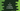# C program to find if a number is a Deficient number or not## C program to find if a number is a Deficient number or not:

Let’s write a program that will check if a given number is a Deficient number or not in C programming language. You will learn what is a deficient number, how to take user inputs in C, how to use loops, how to use functions and how to do simple mathematical calculations.

The program will take a number as input from the user, check if it is a deficient number or not and print a message to the user.

### What is a deficient number:

A number is called a deficient number or defective number if the sum of divisors of the number is less than the value of 2 * number.

Also, the sum of all proper divisors of a deficient number is less than the number itself.

### Example of deficient number:

Let me give you an example. 16 is a deficient number. Its proper divisors are 1, 2, 4 and 8. The sum of these numbers is 1 + 2 + 4 + 8 = 15 which is smaller than 16.

Similarly, 8 is a deficient number because the sum of its proper divisors 1 + 2 + 4 = 7 is smaller than 8.

28 is not a deficient number because the sum of its proper divisors 1 + 2 + 4 + 7 + 14 = 28 is equal to 28, not smaller than 28.

### Algorithm to use to find if a number is a Deficient number:

We will use the below algorithm to find if a number is a deficient number or not:

• Take the number as an input from the user. Store it in a variable.
• Initialize a variable as 0 to hold the sum of all proper divisors.
• Run a loop from 1 to number - 1.
• On each iteration of the loop, check if the current value is a proper divisor of the user given number or not.
• If it is a proper divisor, add it to the sum variable. Else, skip this step.
• Once the loop is completed, the sum variable will hold the sum of all proper divisors of that number.
• Compare the sum with the number. If it is smaller than the number, it will print it as a deficient number. Else, it is not a deficient number.

### C program:

Let’s write down the C program:

``````#include <stdio.h>

int isDeficient(int no)
{
int sum = 0;

for (int i = 1; i < no; i++)
{
if (no % i == 0)
{
sum += i;
}
}
return sum < no;
}

int main()
{
int no;

printf("Enter a number: ");
scanf("%d", &no);

if (isDeficient(no))
printf("%d is a Deficient number.\n", no);
else
printf("%d is not a Deficient number.\n", no);

return 0;
}``````

Here,

• The main() method runs first when you run this program.
• It asks the user to enter a number.
• The number is stored in the integer variable no.
• It calls the isDeficient function and passed the no to this function. If this function returns 1, it prints that the number is a deficient number. Else, it prints that it is not a deficient number.
• The isDeficient function takes a number as the parameter. It returns an integer value, 1 if the given number is deficient, else it returns 0.
• The sum variable is initialized as 0 to hold the sum of all proper divisors.
• The for loop runs from i = 1 to i = no - 1.
• On each step of the loop, it checks if the current value of i is a proper divisor of the number or not. We are using the modulo operator, % to check that. If the value of no%i is 0, i is a proper divisor of no.
• If the current value of i is a proper divisor of the number, add it to the sum variable.
• After the loop is completed, check if the value of sum is smaller than no or not. If yes, return 1, else return 0.

If you run this program, it will give output as like below:

``````Enter a number: 28
28 is not a Deficient number.

Enter a number: 26
26 is a Deficient number.

Enter a number: 2
2 is a Deficient number.``````

## Method 2: Reduce the iteration in the for loop:

We can also reduce the number of iteration in the for loop. Instead of iterating from 1 to number - 1, we can also iterate from 1 to number/2. Because no number larger than number/2 is a proper divisor for a number.

Let’s re-write the program with this change:

``````#include <stdio.h>

int isDeficient(int no)
{
int sum = 0;

for (int i = 1; i <= no / 2; i++)
{
if (no % i == 0)
{
sum += i;
}
}
return sum < no;
}

int main()
{
int no;

printf("Enter a number: ");
scanf("%d", &no);

if (isDeficient(no))
printf("%d is a Deficient number.\n", no);
else
printf("%d is not a Deficient number.\n", no);

return 0;
}``````

The only line we changed is the for loop. It runs from 1 to number/2.

If you run this program, it will print similar output:

``````Enter a number: 28
28 is not a Deficient number.

Enter a number: 27
27 is a Deficient number.``````